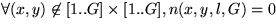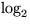Next: Modeling User Choice Up: Security of the DAS Previous: Security of the DAS

### The Size of the Password Space

First we consider the raw size of the password space, or in other words, its information content assuming users are equally likely to pick any element as their password. The raw size is an upper bound on the information content of the distribution that users choose in practice. We need some way to delimit the password space in order to obtain a finite answer, or in probabilistic terms, a way to ascribe probability zero to an infinite subset of passwords, leaving a finite subset that we will count. We assume that all passwords of total length (as defined in Section 3.1) greater than some fixed value have probability zero. We compute the sizeof the space of passwords of total length less than or equal toon a grid of size.is defined in terms of the number of passwords with total length equal to L, P(L,G) by:In turn, P(L,G) can be defined in terms of N(l,G), the number of strokes of length equal to l by:That is, a new stroke of length l may be added to any shorter password of length L-l to make a password of total length L. By defining P(0,G)=1, we complete the definition of the recurrence, once we have given an expression for N(l,G).

The following recurrence relation defines N(l,G). Let n(x,y,l,G) be the number of strokes of length l ending at the cell (x,y) in a grid of size. Then N can be defined in terms of n byClearly,. Moreover, it is convenient to define. The function n can then be evaluated using the following recurrence:

#### n(x,y,l,G) = n(x-1,y,l-1,G) + n(x+1,y,l-1,G) + n(x,y-1,l-1,G) + n(x,y+1,l-1,G)

Putting the pieces together, we can calculate the size of the password space. The results for different upper bounds on total password length on agrid are given in Table 1.

 1 2 3 4 5 6 7 8 9 10(# passwords) 5 10 14 19 24 29 33 38 43 4811 12 13 14 15 16 17 18 19 20(# passwords) 53 58 63 67 72 77 82 87 91 96

The data in Table 1 shows that the raw size of the graphical password space surpasses that of textual passwords for reasonable password configurations. While these numbers are encouraging, in practice not all graphical passwords are equally likely to be chosen by users, rendering a uniform distribution overly optimistic. For example, although the number of passwords of length greater than or equal to 12 is already greater than the number of textual passwords of 8 characters or less constructed from the printable ASCII codes (), this includes all possible combinations of twelve isolated dots.

In order to obtain a more realistic estimate of the information content, in the following section we suggest a model in which we characterize passwords as being memorable'' in terms of the programs which generate them.Next: Modeling User Choice Up: Security of the DAS Previous: Security of the DAS